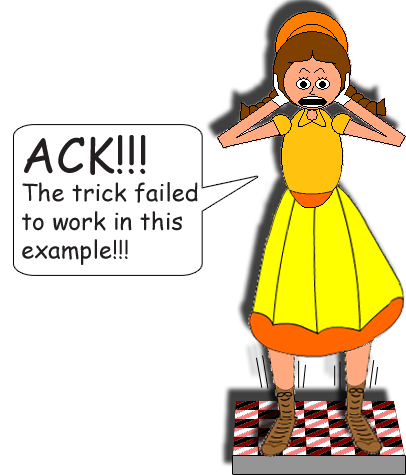# Delightful Division 4

## Examples:

If 4x = 5, then x = 5/4 or 1 + 1/4; If 4 = 5x, then x = 4/5

If 7x = 8, then x = 8/7 or 1 + 1/7; If 7 = 8x, then x = 7/8

If 12x = 84, then x = 7; If 12 = 84x, then x = 1/7

If x√(2) = π, then x = π/√(2) or π√(2)/2; If √(2) = πx, then x = √(2)/π

This math trick works with any 2 numbers, except zero(0) because division by zero(0) is undefined. Any number multiplied by zero(0) is still zero(0), zero(0) divided by any other number is still zero(0), but watch what happens in the counterexample below:

If 25x = 0, then x = 0; If 25 = 0x, then... Wait a minute, there's no solution because the sides aren't equal!If the 2 numbers on both sides of the equal sign are the same, then x = 1; simple as that!

If 9x = 9, then x = 1; If 9 = 9x, then x = 1

If 0x = 0, then x is indeterminate!; If 0 = 0x, then x is indeterminate!

Oops! I almost forgot! If both of those numbers are zero(0), then x is indeterminate, meaning that the value can be anything you want to be! But according to math, an algebra problem is supposed to have a determinate solution.